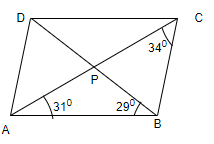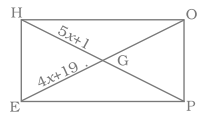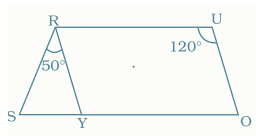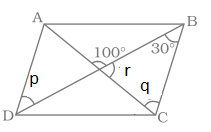In this page we have Understanding quadrilaterals class 8 worksheets with Answers.This worksheet includes Multiple choice question , fill in the blank,True and false, Long answer type questions. Hope you like them and do not forget to like , social share and comment at the end of the page.

Question 1.
Find the number of sides of a regular polygon in which each exterior angle has a measure of 40°$Question 2. The perimeter of the parallelogram is 38 cm . One of the side is 12 cm, find the other side Question 3. Diagonals of the rhombus is 10 cm and 16 cm . What is the area of the rhombus Question 4. One of the angle in the parallelogram is 50°. Find the other angles in the parallelogram Question 5. In the below figure, the measure of the angle DBCQuestion 6. Three angles of a quadrilateral are 50°, 40° and 123°.Find its fourth angle. Question 7. In the figure, HOPE is a rectangle. Its diagonals meet at G. If HG = 5x + 1 and EG = 4x + 19, find xQuestion 8. In the given parallelogram YOUR,$\angle RUO = 120^0$and OY is extended to point S such that$\angle SRY = 50^$. Find$\angle YSR$Question 9. The diagonals of a rhombus are 8 cm and 6 cm. Find its side. Question 10. Two angles of a quadrilateral are each of measure 75° and the other two angles are equal. What is the measure of these two angles ## Fill in the Blank Question 11. (i) Diagonals of a rectangle are __________. (ii)In an parallegram, adjacents angles are _____ (iii)The sum of all exterior angles of a polygon is __________. (iv)The measure of each exterior angle of a regular pentagon is __________. (v) The number of sides of a regular polygon whose each interior angle is of 135° is ___ ## True and False Question 12. (i) Diagonals of a rectangle are perpendicular to each other (ii)All angles of a trapezium are equal (iii)Every square is a trapezium. (iv)If diagonals of a quadrilateral are equal, it must be a rectangle. (v) Every square is a rhombus (vi) ALl parallelograms are kites but all kites are not parallelogram ## Multiple Choice questions Question 13. The sum of angles of a concave quadrilateral is (a) more than 360° (b) less than 360° (c) equal to 360° (d) twice of 360° Question 14. The sum of adjacent angles of a parallelogram is (a) 180° (b) 120° (c) 360° (d) 90° Question 15. ABCD is a rectangle .F and E are the mid points of BC and CD respectively. If area of the$\Delta BEF=7cm^2\$, then the area of the rectangle ABCD is
(a) 49 cm2
(b) 42 cm2
(c) 56 cm2
(d) 28 cm2
Question 16.
Length of one of the diagonals of a rectangle whose sides are 24 cm and 7 cm is
(a) 25 cm
(b) 20 cm
(c) 26 cm
(d) 35 cm
Question 17.
Which of the following can never be the measure of exterior angle of a regular polygon?
(a) 30°
(b) 36°
(c) 45°
(d) 22°
Question 18.ABCD is a parallelogram. Find the value of p
(a) 30°
(b) 100°
(c) 60°
(d) 80°
Question 19.
Find the value of q
(a) 30°
(b) 36°
(c) 45°
(d) 70°
Question 20.
Find the value of r
(a) 30°
(b) 100°
(c) 60°
(d) 80°

## Summary

This Understanding quadrilaterals class 8 worksheets with Answers is prepared keeping in mind the latest syllabus of CBSE . This has been designed in a way to improve the academic performance of the students. If you find mistakes , please do provide the feebback on the mail.